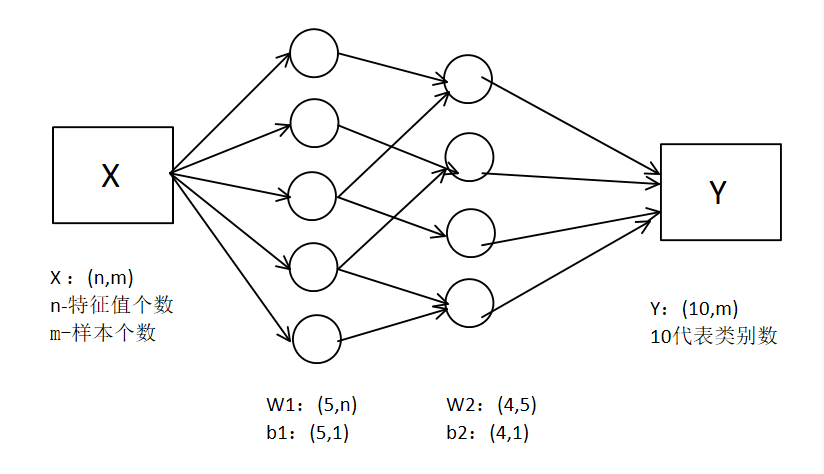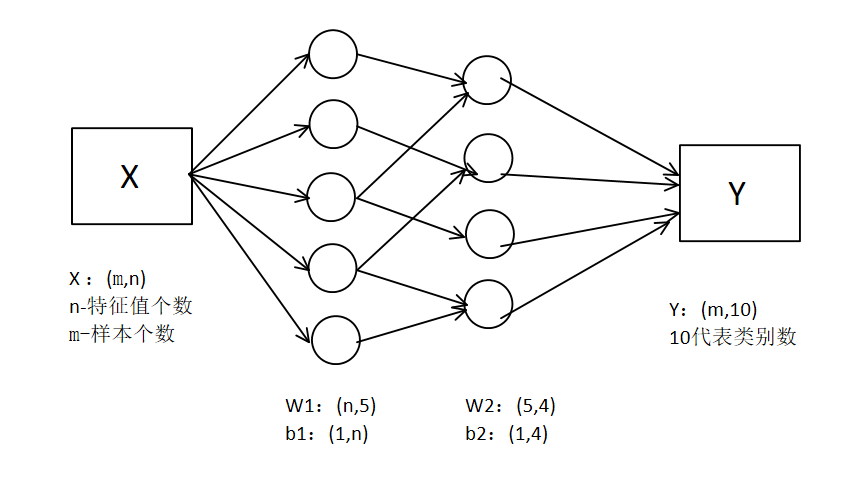# 1.获取数据集

one-hot的意思就是，输出值y应该是一个这样的形式【0,1,2,3,4,5,6,7,8,9】，每一个位置对应一个数字，得到的预测值只有一位为1，其他都是0，也就是说，如果预测值是【1,0,0,0,0,0,0,0,0,0】代表这个数字为0。预测值和给定的y值都是这种形式

# 2.softmax

softmax是一个激活函数，用在多分类问题的最后一层，最后一层使用这个函数以后得到的yhat并不是最终的预测值，以数据集分类例子来说，他是由10个小数组成的向量，并且这10个数的和为1，这10个数分别代表了最后这张图片是哪个数字的几率。选择最大的一项作为1，其他项都为0，最终得到预测值。

# 3.关于数据维度的问题# 4.代码

import tensorflow as tf
#mnist是tensorflow中一个实例，使用input_data来下载/引入数据
from tensorflow.examples.tutorials.mnist import input_data

#获取所有的数据，包括train-set test-set
mnist = input_data.read_data_sets('MNIST_data',one_hot = True)

Extracting MNIST_data\train-images-idx3-ubyte.gz
Extracting MNIST_data\train-labels-idx1-ubyte.gz
Extracting MNIST_data\t10k-images-idx3-ubyte.gz
Extracting MNIST_data\t10k-labels-idx1-ubyte.gz

#训练数据的样本个数
mnist.train.num_examples  #55000

#看看训练集x和y的维度，其中images为X ，labels为Y
print(mnist.train.images.shape)   #(55000, 784)
print(mnist.train.labels.shape)   #(55000, 10)

#测试集
print(mnist.test.images.shape)   #(10000, 784)
print(mnist.test.labels.shape)   #(10000, 10)

#定义批次和一共有多少批次
batch_size = 100
n_batch = mnist.train.num_examples // batch_size

#定义两个占位符
#占位符就是，它并没有真实的数据，但给了下面代码使用数据的机会，等在session中再通过feed-dict来把数据喂给模型
x = tf.placeholder(tf.float32,[None,784])
y = tf.placeholder(tf.float32,[None,10])

#向前传播
w = tf.Variable(tf.zeros([784,10]))
b = tf.Variable(tf.zeros([1,10]))
prediction = tf.nn.softmax(tf.matmul(x,w)+b)

#计算代价值
cost = tf.reduce_mean(tf.square(y-prediction))

#以梯度下降的方式，目标是减少cost值训练一个神经网络 学习因子为0.2

#预测计算准确度
#equal比较两个参数大小是否一样，一样返回true，不一样是false ，得到的其实是true和false的向量
#argmax求y中最大值在哪个位置，1表示对哪个维度找最大值，1表示对应10的那一维
correct_prediction = tf.equal(tf.argmax(y,1),tf.argmax(prediction,1))

#把预测值转化为浮点 ，true变成1.0 false变成0.0 ，再求平均值，
accuracy = tf.reduce_mean(tf.cast(correct_prediction,tf.float32))

#开始训练
with tf.Session() as sess:
#前面定义的变量，就需要先初始化变量
sess.run(tf.global_variables_initializer())

for i in range(100): #迭代100次
for batch in range(n_batch):  #分批次迭代
#获取本批次的数据
batch_xs,batch_ys = mnist.train.next_batch(batch_size)
sess.run(train,feed_dict={x:batch_xs,y:batch_ys})

#每迭代一次使用test集测试一下准确度
acc = sess.run(accuracy,feed_dict={x:mnist.test.images,y:mnist.test.labels})
print('after ',i, 'the accuracy is ',acc)

after  0 the accuracy is  0.8299
after  10 the accuracy is  0.9061
after  20 the accuracy is  0.9133
after  31 the accuracy is  0.9188
after  40 the accuracy is  0.9197
after  50 the accuracy is  0.9212
after  60 the accuracy is  0.9234
after  70 the accuracy is  0.9241
after  80 the accuracy is  0.9243
after  90 the accuracy is  0.9257
after  99 the accuracy is  0.9258

# 5.后记

### 关于代价函数：

tf中也有内置函数可以直接实现交叉熵的计算：

cost = tf.reduce_mean(tf.nn.softmax_cross_entropy_with_logits(labels = y,logits = prediction))

# 使用drop_out：

#drop_our初始化参数 为1.0代表所有的神经元都是工作的
#stddev表示数据的方差为0.1
w1 = tf.Variable(tf.truncated_normal([784,2000],stddev = 0.1))
b1 = tf.Variable(tf.zeros([1,2000])+0.1)
A1 = tf.nn.tanh(tf.matmul(x,w1)+b1)
A1_drop = tf.nn.dropout(A1,keep_prob)

w2 = tf.Variable(tf.truncated_normal([2000,20],stddev = 0.1))
b2 = tf.Variable(tf.zeros([1,20])+0.1)
A2 = tf.nn.tanh(tf.matmul(A1_drop,w2)+b2)
A2_drop = tf.nn.dropout(A2,keep_prob)

w3 = tf.Variable(tf.truncated_normal([20,10],stddev = 0.1))
b3 = tf.Variable(tf.zeros([1,10])+0.1)
A3 = tf.nn.tanh(tf.matmul(A2_drop,w3)+b3)
A3_drop = tf.nn.dropout(A3,keep_prob)

w4 = tf.Variable(tf.truncated_normal([10,10],stddev = 0.1))
b4 = tf.Variable(tf.zeros([1,10])+0.1)
prediction = tf.nn.softmax(tf.matmul(A3_drop,w4)+b4)

sess.run(train,feed_dict={x:batch_xs,y:batch_ys,keep_prob:0.8})

 train_acc = sess.run(accuracy,feed_dict={x:mnist.train.images,y:mnist.train.labels,keep_prob:1.0})
test_acc = sess.run(accuracy,feed_dict={x:mnist.test.images,y:mnist.test.labels,keep_prob:1.0})©️2019 CSDN 皮肤主题: 像素格子 设计师: CSDN官方博客